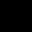# Find (7x + 4y ) / (x-2y) if x/2y = 3/2 ?

Ques:- Find (7x + 4y ) / (x-2y) if x/2y = 3/2 ?
A. 6
B. 8
C. 7
D. data insufficient

## 3 Answers on this Question

1.Admin says:

x/2y=3/2–>x=3.2y/2=>3y
x=3y
7(3y)+4y
……..
3y-2y
25y
….==>25
y

2.Meher Teja says:

Data insufficient

3.DK BOSS says:

x/2y=3/2…
therefore
2x=6y
x=3y
therefor
=21y+4y/3y-2y
=25y/y
=25..